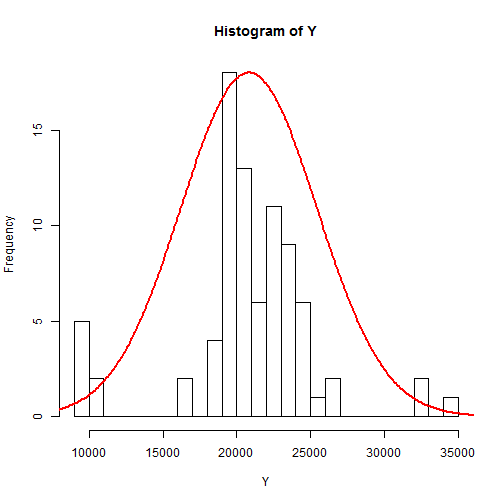# Gibbs sampling for a one sample t-test

### Chapter 3.2.1: Gibbs sampling

Assume $$Y_i|\mu,\sigma^2\sim\mbox{Normal}(\mu,\sigma^2)$$ for $$i=1,…,n$$ and let the prior distributions be $$\mu\sim\mbox{Normal}(0,\sigma^2/m)$$ and $$\sigma^2\sim\mbox{InvGamma}(a,b)$$. It can be shown (Chapter 2) that the full conditional distributions are

$\mu|\sigma^2,Y_1,…,Y_n\sim\mbox{Normal}\left(\frac{\sum_{i=1}^nY_i}{n+m},\frac{\sigma^2}{n+m}\right)$ and $\sigma^2|\mu,Y_1,…,Y_n\sim\mbox{InvGamma}\left(a+n/2,b+\sum_{i=1}^n(Y_i-\mu)^2/2\right).$

Gibbs sampling iterates between drawing from these two (univariate) full conditional distributions to produce samples from the joint (bivariate) posterior distribution.

## Load the galaxy data

 library(MASS)
Y <- galaxies
n <- length(Y)
hist(Y,breaks=25)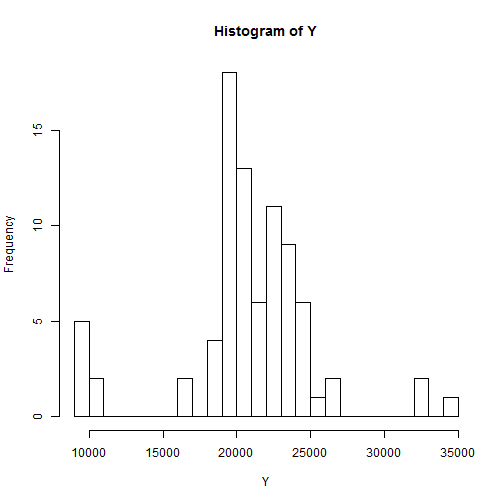## Fix the priors

  m <- 0.01
a <- 0.01
b <- 0.01


## Gibbs sampling

  n.iters <- 30000
keep.mu <- rep(0,n.iters)
keep.s2 <- rep(0,n.iters)

# Initial values
mu         <- mean(Y)
s2         <- var(Y)
keep.mu <- mu
keep.s2 <- s2

for(iter in 2:n.iters){

# sample mu|s2,Y

MN  <- sum(Y)/(n+m)
VR  <- s2/(n+m)
mu <- rnorm(1,MN,sqrt(VR))

# sample s2|mu,Y

A  <- a + n/2
B  <- b + sum((Y-mu)^2)/2
s2 <- 1/rgamma(1,A,B)

# keep track of the results
keep.mu[iter] <- mu
keep.s2[iter] <- s2

# Plot the samples every 10000 iterations
if(iter%%10000==0){
par(mfrow=c(1,2))
plot(keep.mu[1:iter],type="l",ylab="mu")
plot(keep.s2[1:iter],type="l",ylab="s2")
}
}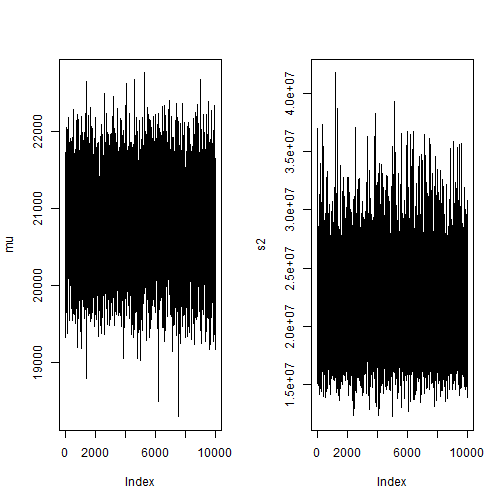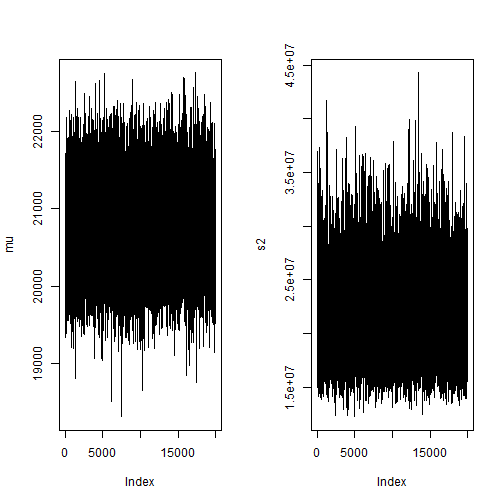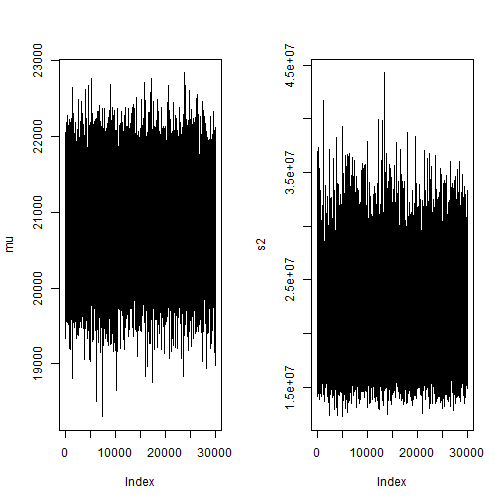## Plot the samples from the joint posterior of $$\mu$$ and $$\sigma^2$$

 plot(keep.s2,keep.mu,xlab="Sigma^2",ylab="mu",main="Joint posterior")
abline(mean(Y),0)
abline(v=var(Y))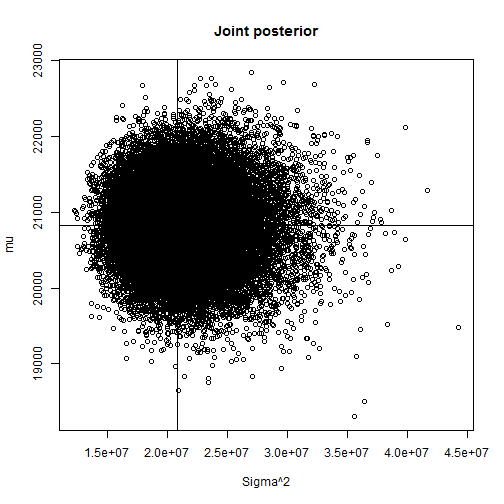## Plot the samples from the marginal (over $$\sigma^2$$) posterior of $$\mu$$, $$p(\mu|Y_1,…,Y_n)$$

 hist(keep.mu,xlab="mu",main="Marginal posterior")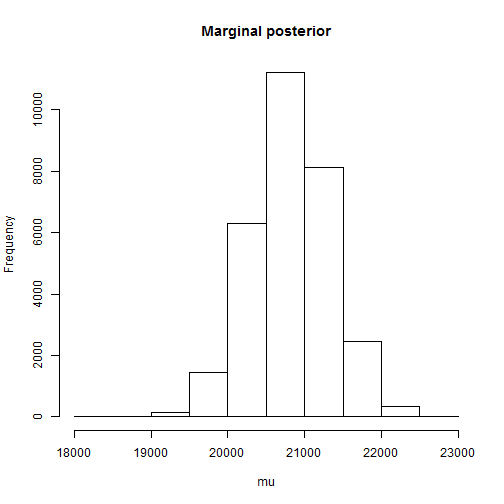## Plot the samples from the marginal (over $$\mu$$) posterior of $$\sigma$$, $$p(\sigma|Y_1,…,Y_n)$$

 keep.s <- sqrt(keep.s2)
hist(keep.s,xlab="sigma",main="Marginal posterior")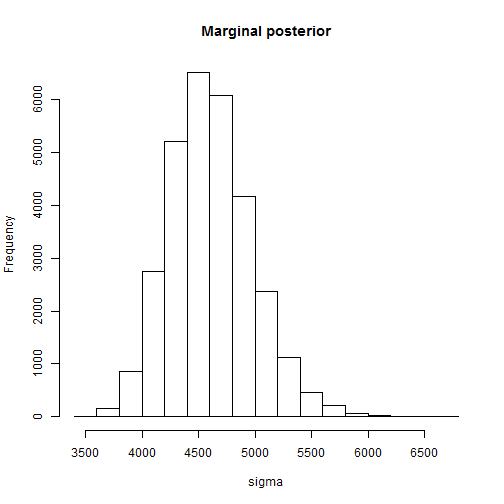## Compute the approximate marginal means and 95\% credible sets

 # mu
mean(keep.mu)

##  20823.19

 quantile(keep.mu,c(0.025,0.975))

##    2.5%   97.5%
## 19827.0 21827.6

 # sigma^2
mean(keep.s2)

##  21380683

 quantile(keep.s2,c(0.025,0.975))

##     2.5%    97.5%
## 15724355 29214747

 # sigma
mean(keep.s)

##  4609.232

 quantile(keep.s,c(0.025,0.975))

##     2.5%    97.5%
## 3965.395 5405.067


## Plot the data versus the fitted model

 mu_hat  <- mean(keep.mu)
sig_hat <- mean(keep.s)
h       <- hist(Y,breaks=25)

y       <- seq(4000,40000,100)
d       <- dnorm(y,mu_hat,sig_hat)
d       <- max(h\$count)*d/max(d)
lines(y,d,lwd=2,col=2)